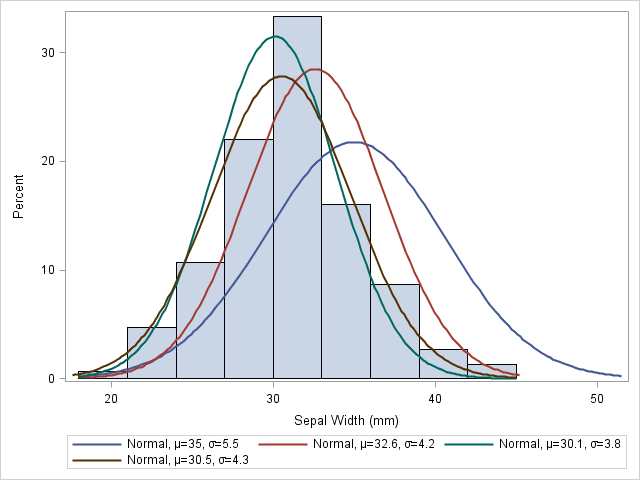# Loss Network

## What is a Loss Network?

A loss network is a neural network that is trained using an optimization process that requires a loss function in order to calculate the model error. The process of optimization relies on the use of an objective function, also known as a cost or loss function because it is used to calculate the model's "loss." The loss function estimates the error of a set of proposed weights in the neural network. In short, the loss function distills the errors of a neural network into a single number such that any improvements in that number are indicative of a better model.

### Maximum Likelihood

A maximum likelihood estimation, or MLE, is used to calculate and the best estimates for parameters from historical training data. The model makes predictions about potential weight values that best maps the inputs to the target output or variable. These predictions are compared to understand how closely the estimated distribution matches the distribution of the target variables. Maximum likelihood employs a principle called "consistency" which state that as the model processes more training data, the estimations that the model produces will become more accurate.Source

### Cross-Entropy

Cross-entropy is the measurement of the error rate produced by the maximum likelihood model. In short, it is used to estimate the differences between an estimated distribution, and a predicted probability distribution. Cross-entropy functions are commonly used as a loss function for classification problems. With regression problems, a mean squared error loss function is a suitable alternative.